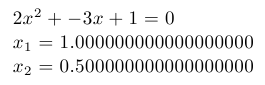# Web2c 2009

Version of implementation Web2c of programming language TeX

Version of Web2c included in TeX Live 2009 distribution.

## Examples:

### Hello, World! - TeX (298):

Hello, World!
\bye


### Quadratic equation - TeX (301):

This example uses TeX fixed point arithmetic package fp and it’s macro for solving quadratic equations called \FPqsolve. Note that this macro can find only real roots of equation, and prints “FP error: Quadratic equation does not have a solution” if roots are complex (i.e., non-existent from its point of view).

\input fp.tex

\message{A = }
\message{B = }
\message{C = }

\FPqsolve{\xone}{\xtwo}{\number\a}{\number\b}{\number\c}

$\a x^2+\b x+\c=0$

$x_1=\xone$

$x_2=\xtwo$
\byeQuadratic equation: document generated by TeX example program

### Factorial - TeX (302):

This example uses iterative factorial definition.

Note that \factorial macro has to use double curly brackets because it has loop that is used inside other loop.

Also only factorials up to 12 are handled. For bigger numbers TeX throws “Arithmetic overflow” error.

\newcount\n \newcount\p \newcount\m

\n=1
\p=1
\loop\ifnum\n<\m \multiply\p by \n \advance\n by 1 \repeat\number\p}}

\n=0
\loop\ifnum\n<\m \hfil\break\number\n! = \factorial{\n} \advance\n by 1 \repeat}

\printfactorials{12}
\bye


### Fibonacci numbers - TeX (303):

This example uses iterative process to calculate Fibonacci numbers.

Note that \fibonacci macro has to use double curly brackets because it has loop that is used inside other loop.

\newcount\n \newcount\np \newcount\npp \newcount\m \newcount\f

\def\fibonacci#1{{\ifnum #1<3 1\else
\np=1\npp=1\m=3
\number\f\fi}}

\n=1

\printfibonacci{16}
\bye